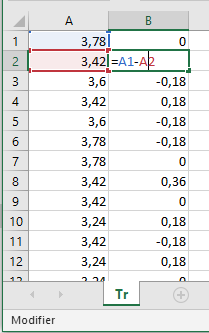# Calculating the change in error!

Hi,
I am searching for something that can help to calculate the change in error (derivative bloc in MATLAB), I can calculate the error but I have no idea how to calculate the change in error! can you help me to figure this out please?

How often do you calculate the error? Can you remember the last error when you calculate the current error? If so, can you subtract the old error from the current error? IF you can, that is the change in error.like this? if so, what would be the first value (0)? since there will be no old error!

Certainly. You are looking for the change in error, so the first one will be the larges change.
Paul

is the 0 value the largest value ?

Probably. Only your tests will tell you that. You are encountering what is known as "initial conditions". They must always be considered in any program.
Paul

This topic was automatically closed 180 days after the last reply. New replies are no longer allowed.